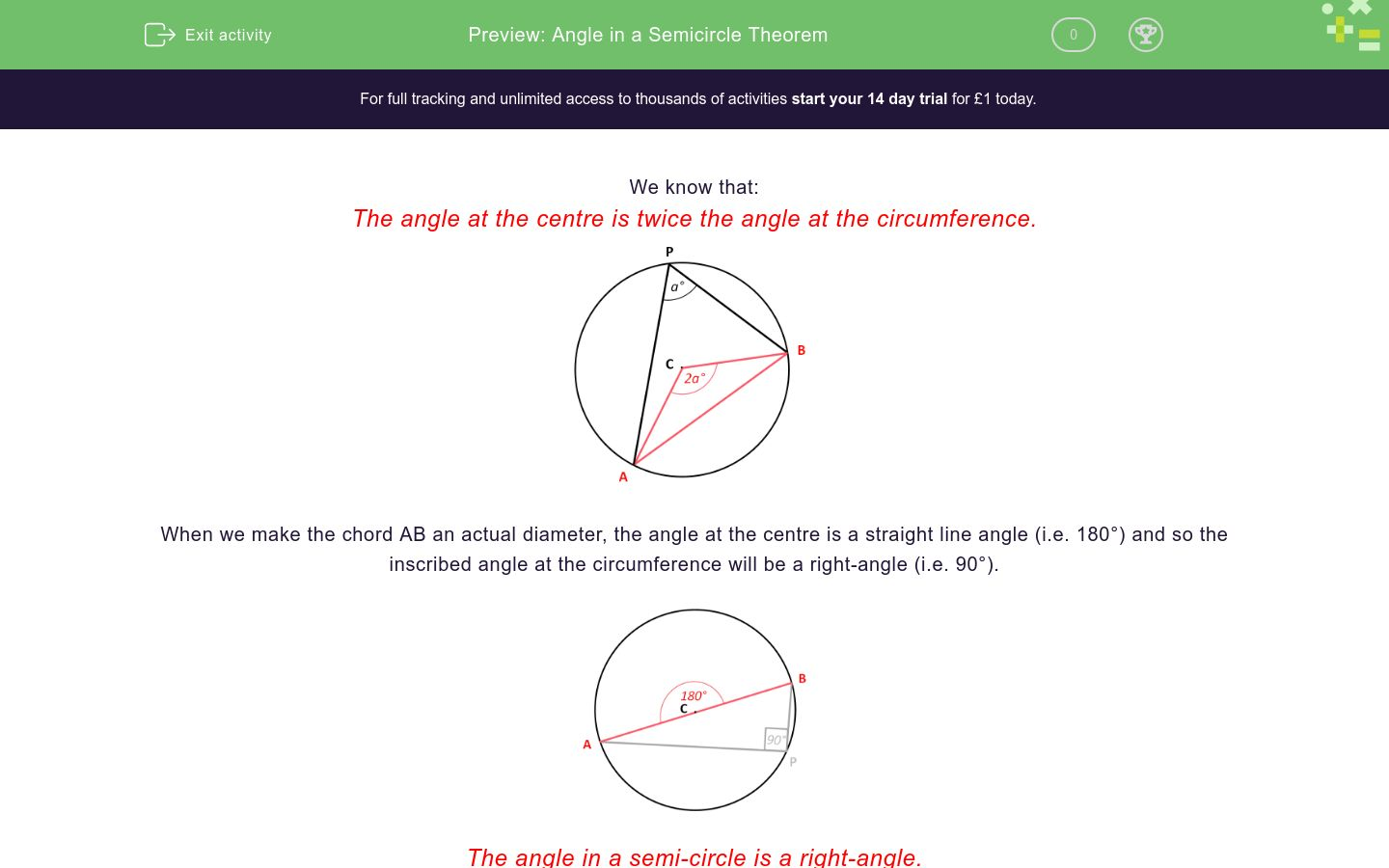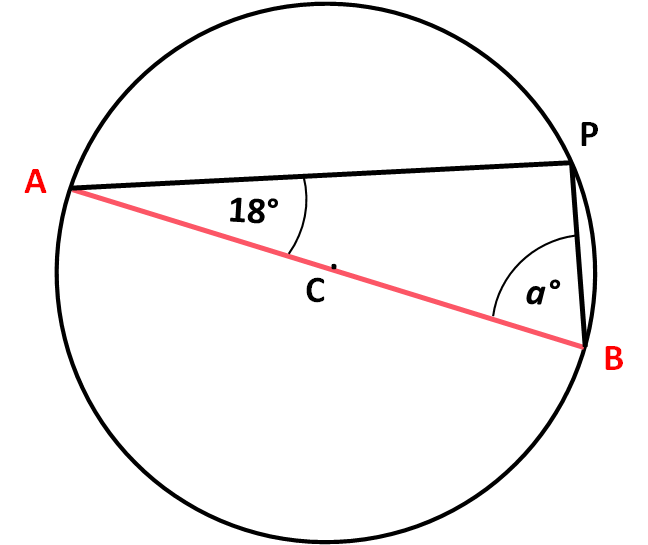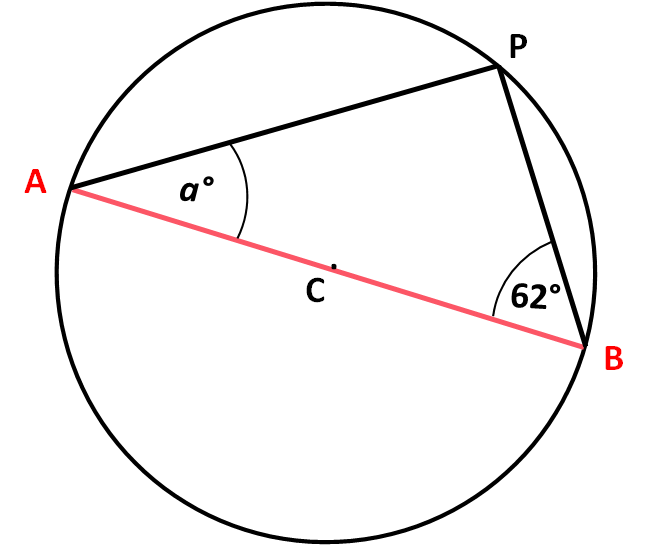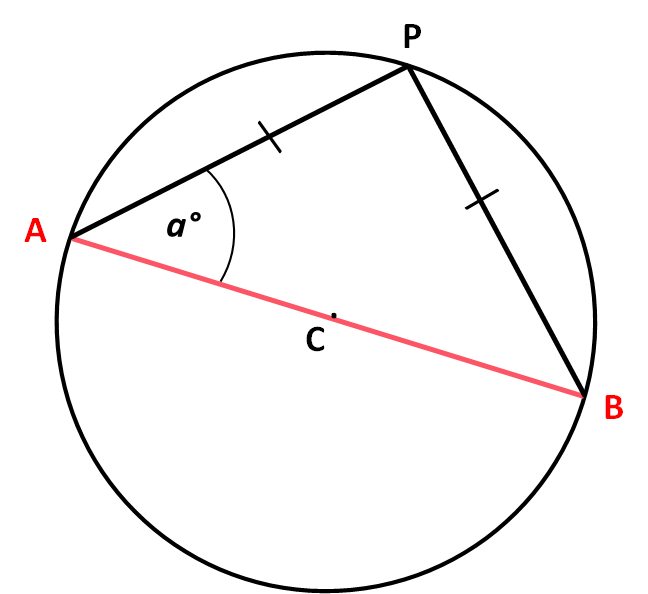# Angle in a Semicircle Theorem

In this worksheet, students must use the rule that an inscribed angle is half the central angle, so the angle in a semicircle is a right-angle.Key stage:  KS 4

Curriculum topic:  Geometry and Measures

Curriculum subtopic:  Identify and Apply Circle Definitions and Properties

Difficulty level:### QUESTION 1 of 10

We know that:

The angle at the centre is twice the angle at the circumference.When we make the chord AB an actual diameter, the angle at the centre is a straight line angle (i.e. 180°) and so the inscribed angle at the circumference will be a right-angle (i.e. 90°).The angle in a semi-circle is a right-angle.

Watch this video to appreciate that the position of the point P does not matter as long as it remains on the circumference.

AB is the diameter of a circle, centre C.

P is a point on the circumference.

Calculate the angle .

Just write the number.AB is the diameter of a circle, centre C.

P is a point on the circumference.

Calculate the angle .

Just write the number.AB is the diameter of a circle, centre C.

P is a point on the circumference.

Calculate the angle .

(Just write the number)AB is the diameter of a circle, centre C.

P is a point on the circumference.

Calculate the angle .

Just write the number.AB is the diameter of a circle, centre C.

P is a point on the circumference.

Calculate the angle .

Just write the number.AB is the diameter of a circle, centre C.

P is a point on the circumference.

Calculate the angle .

Just write the number.AB is the diameter of a circle, centre C.

P is a point on the circumference.

Calculate the angle .

Just write the number.AB is the diameter of a circle, centre C.

P is a point on the circumference.

Calculate the angle .

Just write the number.AB is the diameter of a circle, centre C.

P is a point on the circumference.

Calculate the angle .

Just write the number.AB is the diameter of a circle, centre C.

P is a point on the circumference and AP = BP.

Calculate the angle .

Just write the number.• Question 1

AB is the diameter of a circle, centre C.

P is a point on the circumference.

Calculate the angle .

Just write the number.58
EDDIE SAYS
∠APB = 90°
a° = 180° - 90° - 32°
• Question 2

AB is the diameter of a circle, centre C.

P is a point on the circumference.

Calculate the angle .

Just write the number.61
EDDIE SAYS
∠APB = 90°
a° = 180° - 90° - 29°
• Question 3

AB is the diameter of a circle, centre C.

P is a point on the circumference.

Calculate the angle .

(Just write the number)68
EDDIE SAYS
∠APB = 90°
a° = 180° - 90° - 22°
• Question 4

AB is the diameter of a circle, centre C.

P is a point on the circumference.

Calculate the angle .

Just write the number.18
EDDIE SAYS
∠APB = 90°
a° = 180° - 90° - 72°
• Question 5

AB is the diameter of a circle, centre C.

P is a point on the circumference.

Calculate the angle .

Just write the number.72
EDDIE SAYS
∠APB = 90°
a° = 180° - 90° - 18°
• Question 6

AB is the diameter of a circle, centre C.

P is a point on the circumference.

Calculate the angle .

Just write the number.52
EDDIE SAYS
∠APB = 90°
a° = 180° - 90° - 38°
• Question 7

AB is the diameter of a circle, centre C.

P is a point on the circumference.

Calculate the angle .

Just write the number.28
EDDIE SAYS
∠APB = 90°
a° = 180° - 90° - 62°
• Question 8

AB is the diameter of a circle, centre C.

P is a point on the circumference.

Calculate the angle .

Just write the number.25
EDDIE SAYS
∠APB = 90°
a° = 180° - 90° - 65°
• Question 9

AB is the diameter of a circle, centre C.

P is a point on the circumference.

Calculate the angle .

Just write the number.49
EDDIE SAYS
∠APB = 90°
a° = 180° - 90° - 41°
• Question 10

AB is the diameter of a circle, centre C.

P is a point on the circumference and AP = BP.

Calculate the angle .

Just write the number.45
EDDIE SAYS
∠APB = 90°
ΔAPB is isosceles and ∠PAB = ∠ PBA
a° = ½(180° - 90°)
---- OR ----

Sign up for a £1 trial so you can track and measure your child's progress on this activity.

### What is EdPlace?

We're your National Curriculum aligned online education content provider helping each child succeed in English, maths and science from year 1 to GCSE. With an EdPlace account you’ll be able to track and measure progress, helping each child achieve their best. We build confidence and attainment by personalising each child’s learning at a level that suits them.

Start your £1 trial